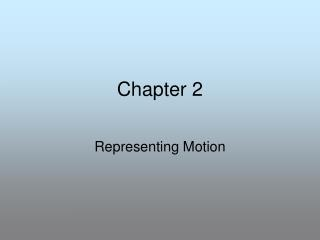DownloadDownload PresentationChapter 2

# Chapter 2

Download Presentation## Chapter 2

- - - - - - - - - - - - - - - - - - - - - - - - - - - E N D - - - - - - - - - - - - - - - - - - - - - - - - - - -
##### Presentation Transcript

1. Chapter 2 Representing Motion

2. Motion diagram- a series of images that show the position of an object at specific time intervals

3. Particle model- a simplified version of the motion diagram in which the images are replaced by single points.

4. When representing motion 2 types of quantities will be used: • Scalars- Quantities that describe only size or magnitude, they are just numbers without a specific direction. Examples would be distance 87 km, 23 m, 400 ft • Vectors- Quantities that describe both magnitude and direction; examples 87 km east, 23m WSW, 400 ft south • Vectorsare represented by arrows that are drawn to scale; When doing a problem involving vectors choose a scale that will produce reasonable vectors (5-10 cm)

5. 80 m/s NE 120 m/s SE 66 m/s W N 8 cm 6.6 cm W E 12 cm Scale 1 cm = 10 m S

6. Resultant vectors-the some of 2 or more vectors • A boy travels .5 km E to a store to pick up bread and milk. The boy continues .2 km E to deliver the milk and bread to his grandmother. What’s the scale? 1cm=.1km 5 cm 2 cm How far did the boy travel? .7 km 7 cm

7. Time intervals and displacements • Two things are used to analyze motion. • Time Intervals- the difference between 2 times Δt= tf - ti Time Interval = final time - initial time • Displacement- the change in position of an object Δd= df – di Displacement = final position minus initial position

8. Distance vs. Displacement • Distance and displacement are not necessarily the same thing. • Distance - How far you travel regardless of direction. • Displacement -"Change in position” this not only takes into account distance but direction as well

9. Example Suppose a person moves in a straight line from the lockers (at a position di = 1.0 m) toward the physics lab (at position = 9.0 m) , as shown below 1.0 m 9.0 m Δd= df – di = 9.0m – 1.0m = 8.0m

10. In this example the distance would be described as 8.0 m, but the displacement would be described as 8.0 m to the right Suppose the person turns around! Δd= df – di = 1.0m – 9.0m = -8.0m The answer is negative so the person must have been traveling horizontally to the left

11. What is the DISPLACEMENT for the entire trip? 0 m is the displacement because you have ended at the same spot What is the total DISTANCE for the entire trip? The distance traveled is 16m 8m + 8m= 16m

12. Position-Time Graphs • Graph used to show the position of an object at a specific time. • Y axis- displacement or position • X-axis- time

13. Where is the object located at 3s? At what point is the object at 7m? What is happening to the object between 6-11s ? What is happening between 11-17s ?

14. Velocity- the speed of an object in a specific direction; it is a vector quantity because it describes magnitude (how fast) and direction • Speed- is simply how fast something is moving; It is a scalar quantity because there is no direction associated with it

15. Average Velocity Velocity is defined as: “The RATE at which DISPLACEMENT changes”. d d d If you are given a Displacement vs. Time graph, to find the velocity of an object during specific time intervals simply find the slope.

16. Slope Because velocity is a vector quantity the direction is usually described as being in a positive or negative (backwards) direction d d d

17. What is the average velocity of the object between 6-11s? 0 m/s object is stopped What is the average velocity of the object between 15-17s? -1.5 m/s in a negative direction What is the average velocity of the object between 0-6s? 1.7 m/s in a positive direction

18. Average Speed - the average speed of an object does not indicate direction therefore is equal to the absolute value of average velocity Instantaneous velocity – the speed and direction of an object at a specific time. Instantaneous speed – the speed of an object at a specific time

19. Equations are often more useful than displacement-time graphs; If an object is moving at a constant velocity its graph will be a straight line. y=mx + b d= vt + di dposition/displacement of object v average velocity t time di initial position